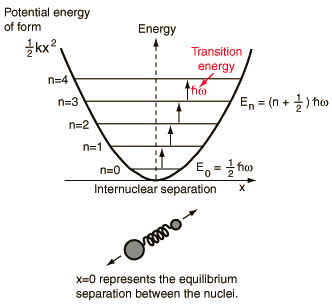# Vibrational Spectra of Diatomic Molecules

The lowest vibrational transitions of diatomic molecules approximate the quantum harmonic oscillator and can be used to imply the bond force constants for small oscillations.The following is a sampling of transition frequencies from the n=0 to n=1 vibrational level for diatomic molecules and the calculated force constants.

 Molecule Frequencyx1013 Hz Force constantN/m HF 12.4* 970 HCl 8.66 480 HBr 7.68 384 HI 6.69 294 CO 6.42 1860 NO 5.63 1550
* From vibrational transition 4138.52 cm-1 in Herzberg's tabulation.

These bond force constants were calculated from the vibrational frequency in the same way the force constant for HCl was calculated.

 Vibration-rotation spectrum of HCl HBr
Index

Schrodinger equation concepts

Molecular spectra concepts

References
Thornton & Rex
Sec 11-1
Barrow

 HyperPhysics***** Quantum Physics R Nave
Go Back